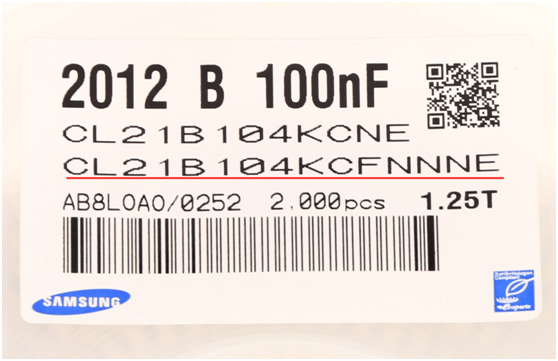## 三星贴片电容的命名方式

三星贴片电容，因其规格齐全，价格适中等良好的口碑，在电子行业中迅速占领了巨大的市场份额，假如你还不知道三星贴片电容的命名方式那么你的工作是难以开展的，下面小编就为大家图文并茂的讲解一下三星贴片电容的命名方式。通常我们直接看第三排上的代码：

规格说明：CL=积层陶瓷电容（简称贴片电容）

21指的是封装为2012

各简称代码如下：

03=0201(0603) 21=0805(2012) 42=1808(4520)

05=0402(1005) 31=1206(3216) 43=1812(4532)

10=0603(1608) 32=1210(3225) 55=2220(5750)

14=0504(1410) 01=0306(0816) 12=0508(1220)

B指的是介质：A=X5R F=Y5V B=X7R X=X6S Y=X7S

104表示的是电容容量

一般电容容量用三位数表示，前面两位为有效数字，第三位为有效数字后"O"的位数

如：104 = 10 0000 (单位pF)

如果中间一位为R 则表示"."

如：3R3 = 3.3pF

K：表示的是误差(B=±0.1pf F=±1% K=±10% C=±0.25pf G=±2% M=±20% D=±0.5pf J=±5% Z=+80/-20% )

C：表示承受的耐压(Q=6.3V P=10V O=16V A= 25V B= 50V C=100V D=200V E=250V G=500V H=630V )

F：表示厚度 (3=0.30毫米 A=0.65毫米 M=1.15毫米 I=2.00毫米 Q=1.25毫米 5=0.50毫米 C=0.85毫米 F=1.25毫米 J=2.50毫米 V=2.50毫米 8=0.80毫米 D=1.00毫米 H=1.60毫米 L=3.20毫米 )

N:表示端头类别（A=常规产品 钯/银/镍屏蔽/锡 100% 、N=常规产品 镍/铜/镍屏蔽/锡 100% 、G=常规产品 铜/铜/镍屏蔽/锡 100% 、L=低侧面产品 镍/铜/镍屏蔽/锡 100% )

N:代表产品( A =阵列(2-元素) B =阵列(4-元素) C=高频 L =LICC N =常规 P =自动 )

E：代表包装方式(B=散装 O=纸版箱料带,10英寸料盘 E=压花纸版箱,7英寸料盘 P=散装箱 D=纸版箱料带,13英寸料盘(10000ea) F=压花纸版箱,13英寸料盘 C=纸版箱料带,7英寸料盘 L=纸版箱料带,13英寸料盘(15,000ea) S=压花纸版箱,10英寸料盘)

以上就是三星贴片电容的命名方式，而各大品牌的命名方式都各有差异，其他品牌电容命名识别请参考其公司规格书。

东莞市佳益电子科技专业销售三星常规贴片电容、高压贴片电容、大容值贴片电容、三星排容、汽车电容、中高压电容、低SEL陶瓷电容以及0402-0603超小尺寸贴片电容，大量原厂现货发售，欢迎询价。联系：400-150-0848。

本文由东莞市佳益电子科技有限公司原创，转载请注明出处!